2019-12-04 00:43:18 qq_35306281 阅读数 27
• ###### 携手STM32CubeMX玩转STM32

783 人正在学习 去看看 李凯龙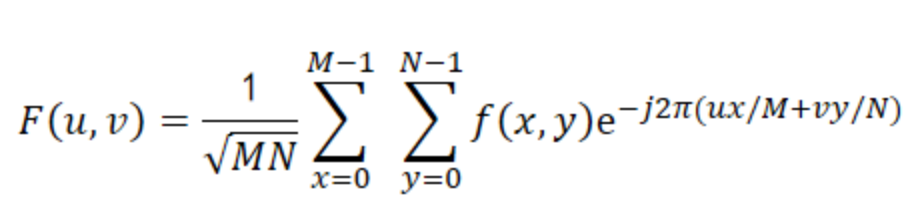逆变换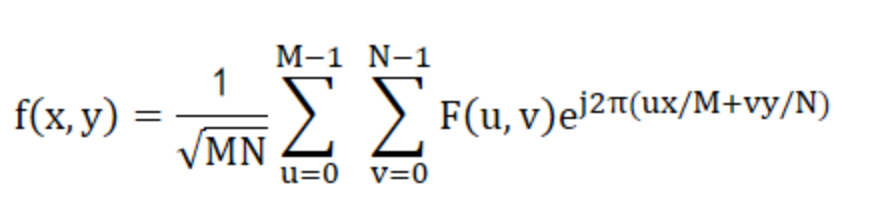``````#include <stdio.h>
#include <complex>
#include <stdlib.h>
#include <vector>
#include <assert.h>
#include <opencv2/opencv.hpp>

#define PI 3.1415926
template<class T>
double static_cast_double(T a)
{
return static_cast<double>(a);
}
template<class T>
float static_cast_float(T a)
{
return static_cast<float>(a);
}
template<class T>
unsigned char static_cast_uchar(T a)
{
return static_cast<unsigned char>(a);
}

class dft{
public:
dft(){};
virtual ~dft(){};
public:
cv::Mat dt(const cv::Mat& src, bool backwards = false);
cv::Mat reduce(const cv::Mat& ft);
protected:
cv::Mat pt(const cv::Mat& src);
cv::Mat bt(const cv::Mat& src);
};

cv::Mat dft::pt(const cv::Mat& src)
{

int M = src.rows;
int N = src.cols;
int u, v, m, n;

std::complex<double> ci{ 0, 1 };
std::vector<float> real_t;
std::vector<float> imag_t;
for (u = 0; u < M; u++)
for (v = 0; v < N; v++)
{
std::complex<double> sum_0(0.0, 0.0);
for (m = 0; m < M; m++)
{
std::complex<double> sum_1(0.0, 0.0);
for (n = 0; n < N; n++)
{
sum_1 += std::exp(-2 * PI*n*v / N*ci) * static_cast_double(src.at<uchar>(m, n));
}
sum_1 /= (double)N;
sum_0 += sum_1 * std::exp(-2 * PI*m*u / M*ci);
}
std::complex<double> tmp = sum_0 / (double)M;
real_t.push_back(static_cast_float(tmp.real()));
imag_t.push_back(static_cast_float(tmp.imag()));
}
cv::Mat s1(real_t);
s1 = s1.reshape(0, M);
cv::Mat s2(imag_t);
s2 = s2.reshape(0, M);
cv::Mat s = { s1, s2 };
cv::Mat dst;
cv::merge(s, 2, dst);
return dst;
}

cv::Mat dft::bt(const cv::Mat& ft)
{
assert(ft.channels() == 2);
int M = ft.rows;
int N = ft.cols;
double M_ = static_cast_double(M);
double N_ = static_cast_double(N);

std::vector<cv::Mat> ft_mats;
cv::split(ft, ft_mats);
int m, n, u, v;
std::complex<double> ci{ 0.0, 1.0 };
std::vector<uchar> real_t;
for (m = 0; m < M; m++)
for (n = 0; n < N; n++)
{
std::complex<double>sum{ 0.0, 0.0 };
for (u = 0; u < M; u++)
for (v = 0; v < N; v++)
{
float rt = ft_mats.at<float>(u, v);
float it = ft_mats.at<float>(u, v);
std::complex<double> temp{ rt, it };
sum += temp * std::exp(2 * PI*(m*u / M_ + n*v / N_));
}
real_t.push_back(static_cast_uchar(sum.real()));
}
cv::Mat real(real_t);
real = real.reshape(0, M);
return real;
}

cv::Mat dft::reduce(const cv::Mat& ft)
{
assert(ft.channels() == 2);
std::vector<cv::Mat> ft_mats;
cv::split(ft, ft_mats);
cv::magnitude(ft_mats, ft_mats, ft_mats);
cv::Mat magnitudeImage = ft_mats;
magnitudeImage += cv::Scalar::all(1);
cv::log(magnitudeImage, magnitudeImage);

//magnitudeImage.convertTo(magnitudeImage, CV_8UC1, 255, 0);

int cx = magnitudeImage.cols / 2;
int cy = magnitudeImage.rows / 2;
cv::Mat q0(magnitudeImage(cv::Rect(0, 0, cx, cy)));
cv::Mat q1(magnitudeImage(cv::Rect(cx, 0, cx, cy)));
cv::Mat q2(magnitudeImage(cv::Rect(0, cy, cx, cy)));
cv::Mat q3(magnitudeImage(cv::Rect(cx, cy, cx, cy)));

cv:: Mat tmp;
q0.copyTo(tmp);
q3.copyTo(q0);
tmp.copyTo(q3);

q1.copyTo(tmp);
q2.copyTo(q1);
tmp.copyTo(q2);

cv::normalize(magnitudeImage, magnitudeImage, 0, 1, cv::NORM_MINMAX);

return magnitudeImage;
}

cv::Mat dft::dt(const cv::Mat& src, bool backwards)
{
return backwards ? bt(src) : pt(src);
}

int main(int argc, char** argv)
{
cv::resize(src, src, cv::Size(src.cols / 8, src.rows / 8));

dft* dftops = new dft();

cv::Mat ft = dftops->dt(src);

ft = dftops->reduce(ft);

cv::imshow("fft.jpg", ft);
cv::waitKey(0);
delete dftops;
return 0;
}
``````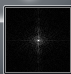2019-04-23 16:24:29 Eastmount 阅读数 8825
• ###### 携手STM32CubeMX玩转STM32

783 人正在学习 去看看 李凯龙

PS：请求帮忙点个Star，哈哈，第一次使用Github，以后会分享更多代码，一起加油。

1.图像傅里叶变换
2.Numpy实现傅里叶变换
3.Numpy实现傅里叶逆变换
4.OpenCV实现傅里叶变换
5.OpenCV实现傅里叶逆变换

PS：文章参考自己以前系列图像处理文章及OpenCV库函数，同时参考如下文献：
《数字图像处理》（第3版），冈萨雷斯著，阮秋琦译，电子工业出版社，2013年.
《数字图像处理学》（第3版），阮秋琦，电子工业出版社，2008年，北京.
《OpenCV3编程入门》，毛星云，冷雪飞，电子工业出版社，2015，北京.

tenderwx-数字图像处理-傅里叶变换在图像处理中的应用

# 一.图像傅里叶变换原理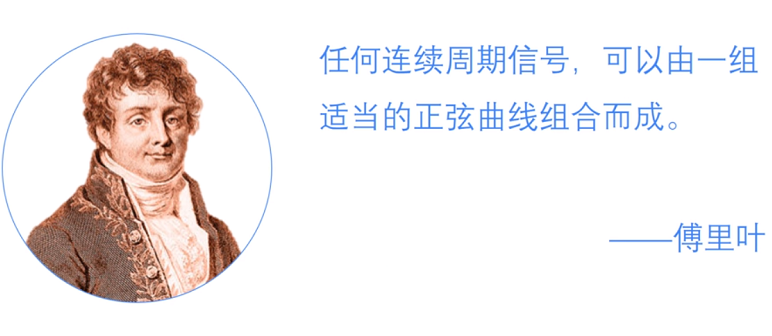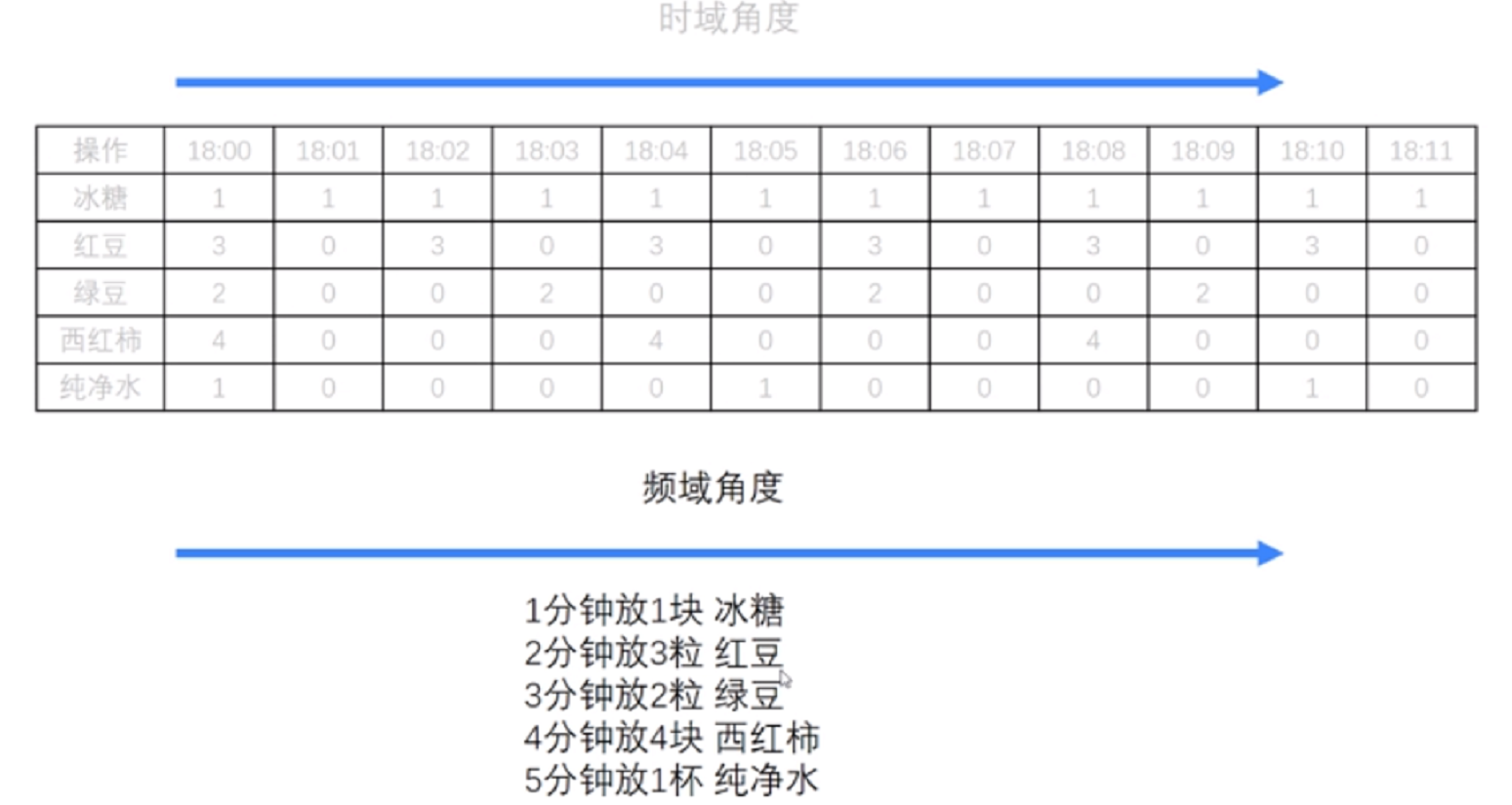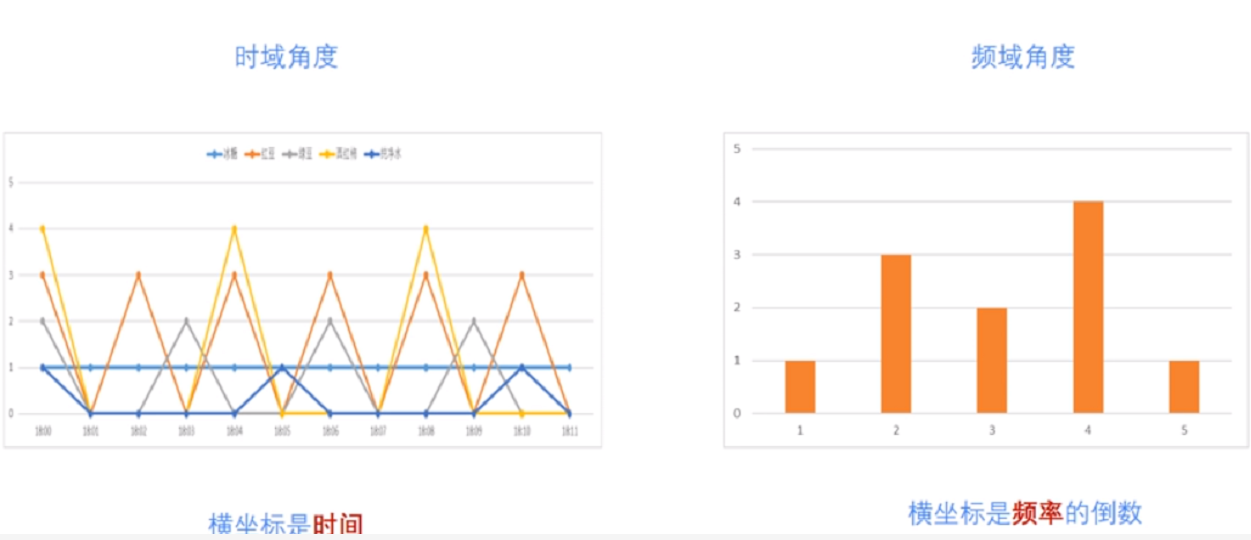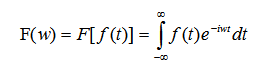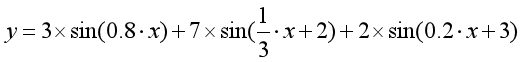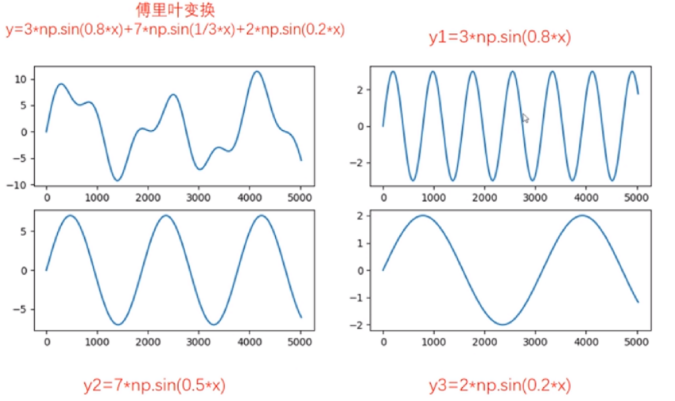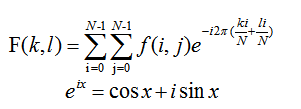# 二.Numpy实现傅里叶变换

Numpy中的 FFT包提供了函数 np.fft.fft2()可以对信号进行快速傅里叶变换，其函数原型如下所示，该输出结果是一个复数数组（Complex Ndarry）。

fft2(a, s=None, axes=(-2, -1), norm=None)

• a表示输入图像，阵列状的复杂数组
• s表示整数序列，可以决定输出数组的大小。输出可选形状（每个转换轴的长度），其中s表示轴0，s表示轴1。对应fit(x,n)函数中的n，沿着每个轴，如果给定的形状小于输入形状，则将剪切输入。如果大于则输入将用零填充。如果未给定’s’，则使用沿’axles’指定的轴的输入形状
• axes表示整数序列，用于计算FFT的可选轴。如果未给出，则使用最后两个轴。“axes”中的重复索引表示对该轴执行多次转换，一个元素序列意味着执行一维FFT
• norm包括None和ortho两个选项，规范化模式（请参见numpy.fft）。默认值为无

Numpy中的fft模块有很多函数，相关函数如下：

#计算一维傅里叶变换
numpy.fft.fft(a, n=None, axis=-1, norm=None)
#计算二维的傅里叶变换
numpy.fft.fft2(a, n=None, axis=-1, norm=None)
#计算n维的傅里叶变换
numpy.fft.fftn()
#计算n维实数的傅里叶变换
numpy.fft.rfftn()
#返回傅里叶变换的采样频率
numpy.fft.fftfreq()
#将FFT输出中的直流分量移动到频谱中央
numpy.fft.shift()

``````# -*- coding: utf-8 -*-
import cv2 as cv
import numpy as np
from matplotlib import pyplot as plt

#读取图像

#快速傅里叶变换算法得到频率分布
f = np.fft.fft2(img)

#默认结果中心点位置是在左上角,
#调用fftshift()函数转移到中间位置
fshift = np.fft.fftshift(f)

#fft结果是复数, 其绝对值结果是振幅
fimg = np.log(np.abs(fshift))

#展示结果
plt.subplot(121), plt.imshow(img, 'gray'), plt.title('Original Fourier')
plt.axis('off')
plt.subplot(122), plt.imshow(fimg, 'gray'), plt.title('Fourier Fourier')
plt.axis('off')
plt.show()
``````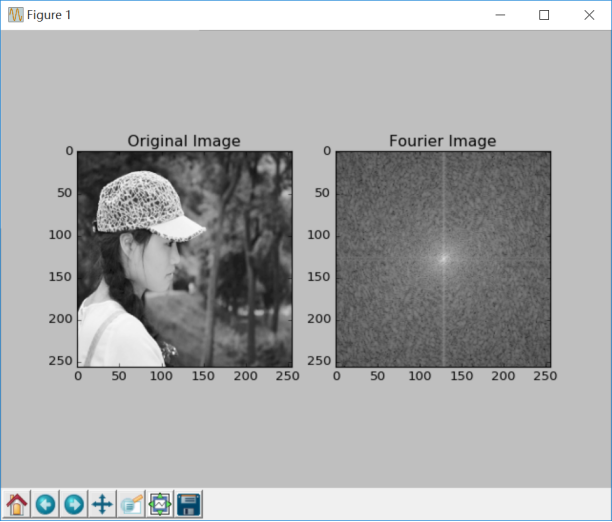# 三.Numpy实现傅里叶逆变换

#实现图像逆傅里叶变换，返回一个复数数组
numpy.fft.ifft2(a, n=None, axis=-1, norm=None)
#fftshit()函数的逆函数，它将频谱图像的中心低频部分移动至左上角
numpy.fft.fftshift()
#将复数转换为0至255范围
iimg = numpy.abs(逆傅里叶变换结果)

``````# -*- coding: utf-8 -*-
import cv2 as cv
import numpy as np
from matplotlib import pyplot as plt

#读取图像

#傅里叶变换
f = np.fft.fft2(img)
fshift = np.fft.fftshift(f)
res = np.log(np.abs(fshift))

#傅里叶逆变换
ishift = np.fft.ifftshift(fshift)
iimg = np.fft.ifft2(ishift)
iimg = np.abs(iimg)

#展示结果
plt.subplot(131), plt.imshow(img, 'gray'), plt.title('Original Image')
plt.axis('off')
plt.subplot(132), plt.imshow(res, 'gray'), plt.title('Fourier Image')
plt.axis('off')
plt.subplot(133), plt.imshow(iimg, 'gray'), plt.title('Inverse Fourier Image')
plt.axis('off')
plt.show()
``````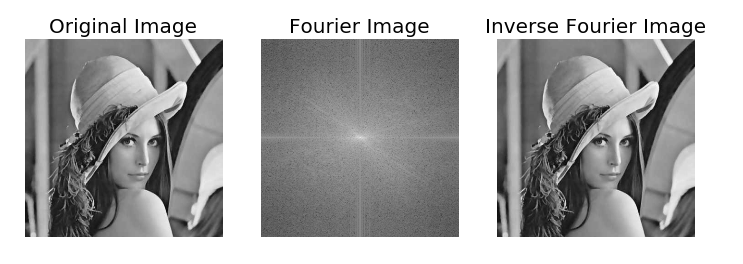# 四.OpenCV实现傅里叶变换

OpenCV 中相应的函数是cv2.dft()和用Numpy输出的结果一样，但是是双通道的。第一个通道是结果的实数部分，第二个通道是结果的虚数部分，并且输入图像要首先转换成 np.float32 格式。其函数原型如下所示：

dst = cv2.dft(src, dst=None, flags=None, nonzeroRows=None)

• src表示输入图像，需要通过np.float32转换格式
• dst表示输出图像，包括输出大小和尺寸
• flags表示转换标记，其中DFT _INVERSE执行反向一维或二维转换，而不是默认的正向转换；DFT _SCALE表示缩放结果，由阵列元素的数量除以它；DFT _ROWS执行正向或反向变换输入矩阵的每个单独的行，该标志可以同时转换多个矢量，并可用于减少开销以执行3D和更高维度的转换等；DFT _COMPLEX_OUTPUT执行1D或2D实数组的正向转换，这是最快的选择，默认功能；DFT _REAL_OUTPUT执行一维或二维复数阵列的逆变换，结果通常是相同大小的复数数组，但如果输入数组具有共轭复数对称性，则输出为真实数组
• nonzeroRows表示当参数不为零时，函数假定只有nonzeroRows输入数组的第一行（未设置）或者只有输出数组的第一个（设置）包含非零，因此函数可以处理其余的行更有效率，并节省一些时间；这种技术对计算阵列互相关或使用DFT卷积非常有用

cv2.magnitude(x, y)

• x表示浮点型X坐标值，即实部
• y表示浮点型Y坐标值，即虚部
最终输出结果为幅值，即：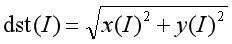``````# -*- coding: utf-8 -*-
import numpy as np
import cv2
from matplotlib import pyplot as plt

#读取图像

#傅里叶变换
dft = cv2.dft(np.float32(img), flags = cv2.DFT_COMPLEX_OUTPUT)

#将频谱低频从左上角移动至中心位置
dft_shift = np.fft.fftshift(dft)

#频谱图像双通道复数转换为0-255区间
result = 20*np.log(cv2.magnitude(dft_shift[:,:,0], dft_shift[:,:,1]))

#显示图像
plt.subplot(121), plt.imshow(img, cmap = 'gray')
plt.title('Input Image'), plt.xticks([]), plt.yticks([])
plt.subplot(122), plt.imshow(result, cmap = 'gray')
plt.title('Magnitude Spectrum'), plt.xticks([]), plt.yticks([])
plt.show()
``````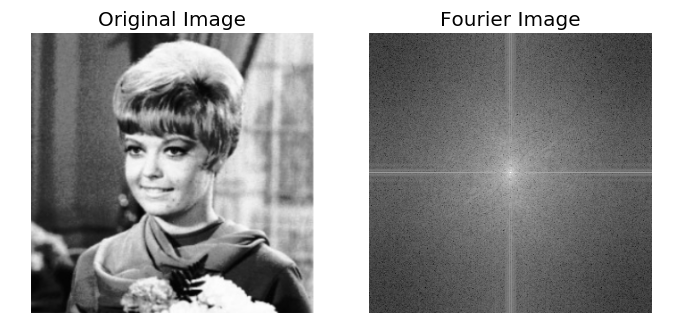# 五.OpenCV实现傅里叶逆变换

dst = cv2.idft(src[, dst[, flags[, nonzeroRows]]])

• src表示输入图像，包括实数或复数
• dst表示输出图像
• flags表示转换标记
• nonzeroRows表示要处理的dst行数，其余行的内容未定义（请参阅dft描述中的卷积示例）

``````# -*- coding: utf-8 -*-
import numpy as np
import cv2
from matplotlib import pyplot as plt

#读取图像

#傅里叶变换
dft = cv2.dft(np.float32(img), flags = cv2.DFT_COMPLEX_OUTPUT)
dftshift = np.fft.fftshift(dft)
res1= 20*np.log(cv2.magnitude(dftshift[:,:,0], dftshift[:,:,1]))

#傅里叶逆变换
ishift = np.fft.ifftshift(dftshift)
iimg = cv2.idft(ishift)
res2 = cv2.magnitude(iimg[:,:,0], iimg[:,:,1])

#显示图像
plt.subplot(131), plt.imshow(img, 'gray'), plt.title('Original Image')
plt.axis('off')
plt.subplot(132), plt.imshow(res1, 'gray'), plt.title('Fourier Image')
plt.axis('off')
plt.subplot(133), plt.imshow(res2, 'gray'), plt.title('Inverse Fourier Image')
plt.axis('off')
plt.show()
``````# 六.总结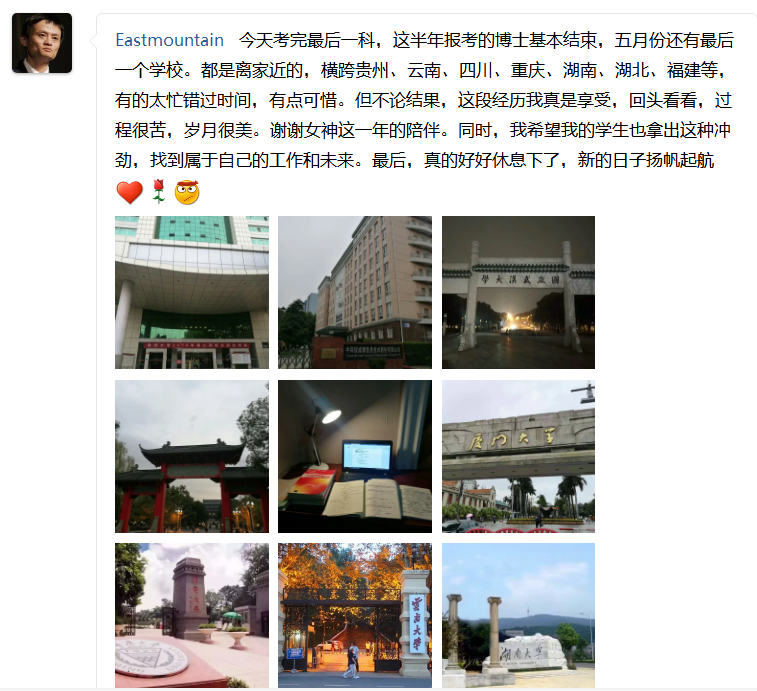（By：Eastmount 2019-04-23 周二下午6点写于花溪 https://blog.csdn.net/Eastmount )

2018-05-03 16:40:43 ssqqssqq 阅读数 1964
• ###### 携手STM32CubeMX玩转STM32

783 人正在学习 去看看 李凯龙

# 分数阶傅里叶变换在信号检测与图像处理中的应用研究（李琼）

## 发展历史

• Wiener等人最早开始研究分数阶傅里叶变换，他对傅里叶变换中的特征值进行了修正，从而使得其比普通傅里叶变换具有更加完善的形式，是分数阶傅里叶变换的最初理论。
• 1937年， Condon 独自研究了分数阶傅里叶变换的基本概念，同时也是第一个直接研究FRFT定义的人;
• 1961年，Bargmann讨论了FRFT的基本定义，并提出FRFT的两种等价的定义形式：Hermit多项式和积分变换;
• 1980年，Namias从特征值域特征函数的角度，重新给出了FRFT的定义，并把FRFT定义为传统傅里叶变换的分数幂形式
• 1993年，Mendlovic，Lohamann和Ozaktas给出了FRFT的光学实现并将其广泛应用于光学领域中，但因缺乏快速算法，始终未受到重视;
• 1993年，Almeida提出FRFT可以解释维时频平面旋转;
• 1996年，Ozaktas提出一种计算量与FFT相当的快速算法以后，FRFT才广泛引起研究者的注意

## 特性

• 将信号从时域变换到时频平面，同时反映信号的时域和频域信息，有利于全面分析信号的局部细微特征
• 是一种线性变换，用分数域中的单一变量表示信号的时频信息且没有交叉项的干扰
• 可看作是信号在时频面上的坐标轴绕原点逆时针转动任意角度后所形成分数域上的表示
• 保留傅里叶变换的优良特性，而且还兼有自身独特的优势
• 线性，旋转相加性，可逆性，酉性，Parseval关系式、Wigner，时移特性，频移特性、尺度特性

## 二维分数阶傅里叶变换

• 先对二维离散信号f的列向量做一维离散FRFT，得到F1
• 对F1的行向量做一维离散FRFT，得到F2
• 对F2转置，得到f的二维离散分数阶傅里叶变换

## 基于分数阶傅里叶变换的图像分析

### 分数阶变换域中图像的能量分布

• 随着p的增大，能量越来越集中，当p=0.7左右，分数域的能量在此中心区域已经达到了90%以上。
• FRFT包含图像的时频信息，随着p的改变，能量在时频域的分配也发生变换，当p<0.5时，将近又50%的能量分散在时域，当p>0.5时，频域能量分布呈明显上升趋势，当p=1时，图像的能量聚集性达到最强。

### 分数阶傅里叶变换域中的图像的幅度和相位信息

• 当阶次较小时（p趋近于0），可以明显看到图片的一些轮廓特征，随着变换阶次 不断增大，纹理信息逐渐减少。表明相位信息所包含的时域信息随着变换阶次的增大而减少，而频域信息随着变化阶次的增大而增大。

## 在图像增强中的应用

### 图像增强

2019-09-28 16:21:13 qq_43294951 阅读数 193
• ###### 携手STM32CubeMX玩转STM32

783 人正在学习 去看看 李凯龙

# 任务要求### 首先是直方图以及均衡化

``````function hist=count_hist(picture) %计算直方图，输入变量为灰度值，返回矩阵为直方图
Sum=zeros(2,256);%第一行表示的是出现的次数，第二行表示的是0~255供给256个灰度值
for i=1:256
Sum(2,i)=i-1;
end   %第二行的初始化过程
for i=1:size(picture,1)
for j=1:size(picture,2)
Sum(1,picture(i,j)+1)=Sum(1,picture(i,j)+1)+1;%由于灰度图像值是0~255，但索引为1~256，所以索引的时候需要编程X(i，j)+1
end
end  %对第一行进行赋值
hist=Sum;
end
``````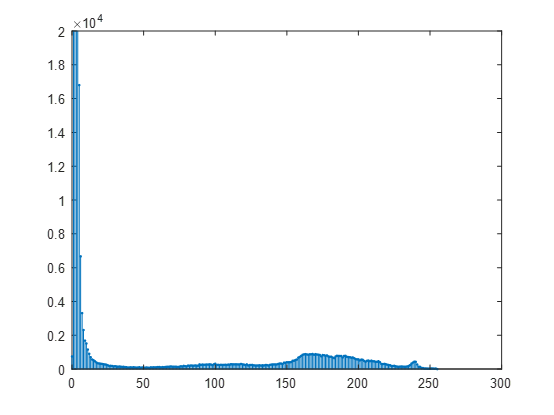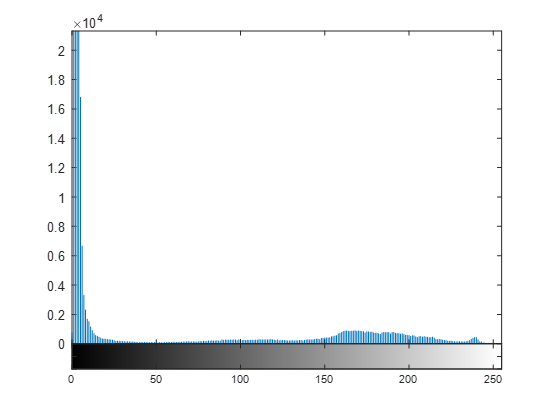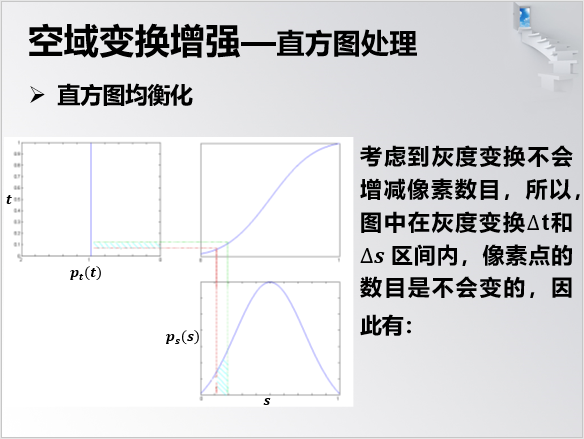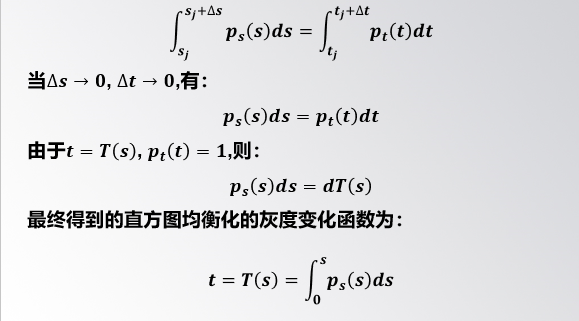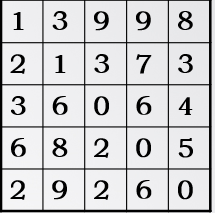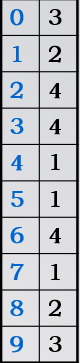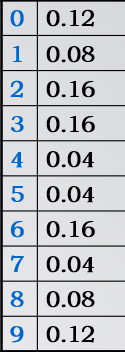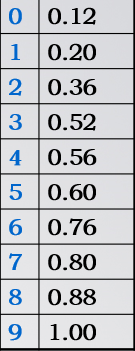HS=[0.12, 0.20, 0.36, 0.52, 0.56, 0.60, 0.76, 0.80, 0.88, 1.00]

HS=[30.6000 51.0000 91.8000 132.6000 142.8000 153.0000 193.8000 204.0000 224.4000 255.0000]

HS=[31 51 92 133 143 153 194 204 224 255]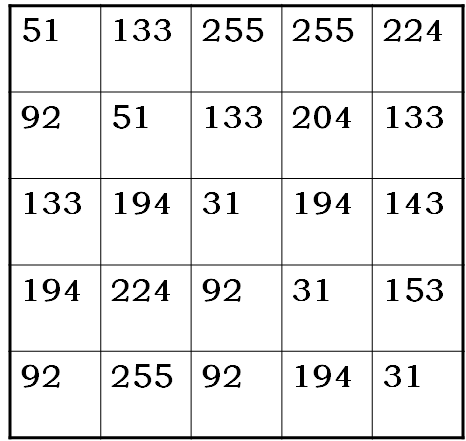``````function [new_picture,new_hist]=hist_equal(picture,hist)%直方图均衡化
Y=hist(1,:)/(size(picture,1)*size(picture,2));%将得到的直方图求每个灰度值所对应的概率
for i=2:length(hist(1,:))
Y(i)=Y(i-1)+Y(i);      %这部分是变成概率密度函数，就是进行概率的累加
end
Y=Y*255;%乘以灰度值255
Y=round(Y);%取整
new_picture=ones(size(picture,1),size(picture,2));%建立新的图像
for i=1:size(picture,1)
for j=1:size(picture,2)
new_picture(i,j)=Y(picture(i,j)+1);   %同样，由于灰度图像值是0~255，但索引为1~256，所以索引的时候需要编程picture(i，j)+1
end
end
new_picture=uint8(new_picture);%由于上面的到的类型是double行，imshow的时候需要unit8型的变量
new_hist=count_hist(new_picture);%均衡化后的直方图
end
``````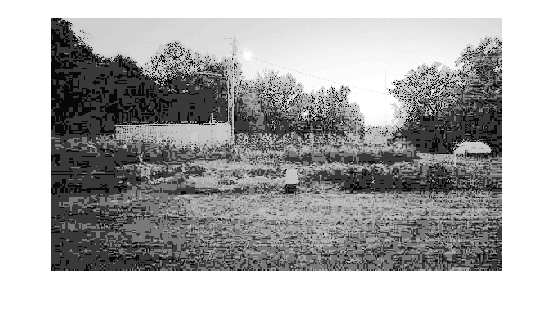## 离散傅里叶变换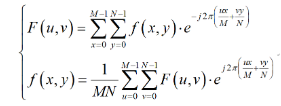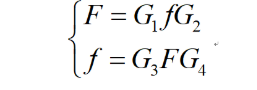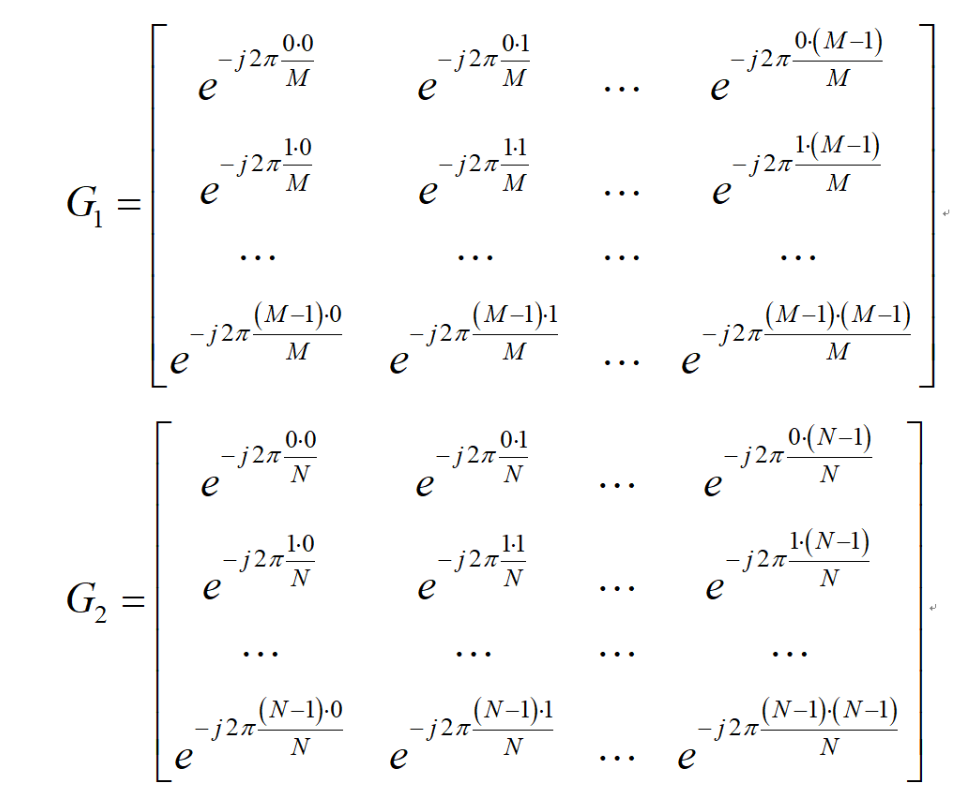G3 G4分别为G1,G2的逆

f(x,y)*(-1)^(x+y)

``````function FFT_picture=FFT(picture)  %变换并且中心化
G1=zeros(size(picture,1),size(picture,1));   %前变换矩阵
G2=zeros(size(picture,2),size(picture,2));   %后变换矩阵
picture1=double(picture);   %将图像uint8格式转换成double型，便于进行复数相乘
for i=1:size(picture,1)
for j=1:size(picture,2)
picture1(i,j)=picture1(i,j)*exp(complex(0,1)*2*pi*(i+j)/2);%对原图像进行中心化预处理
end
end
for i=1:size(picture,1)   %前变换矩阵的赋值
for j=1:size(picture,1)
G1(i,j)=exp(-complex(0,1)*2*pi*(i-1)*(j-1)/size(picture,1));
end
end
for i=1:size(picture,2)   %后变换矩阵的赋值
for j=1:size(picture,2)
G2(i,j)=exp(-complex(0,1)*pi*2*(i-1)*(j-1)/size(picture,2));
end
end
FFT_picture=G1*picture1*G2;   %二维傅里叶变换并且进行了中心化，返回的是复数型矩阵，要是想进行显示...
%需要在程序中运行   imshow(log(abs(FFT_picture)+1),[])
end
``````

``````function IFFT_picture=IFFT(f_picture)  %逆变换并且退中心化
G1=zeros(size(f_picture,1),size(f_picture,1));%前变换矩阵
G2=zeros(size(f_picture,2),size(f_picture,2));%后变换矩阵
for i=1:size(f_picture,1)   %前变换矩阵赋初值
for j=1:size(f_picture,1)
G1(i,j)=exp(complex(0,1)*2*pi*(i-1)*(j-1)/size(f_picture,1))/size(f_picture,1);
end
end
for i=1:size(f_picture,2)  %后变换矩阵赋初值
for j=1:size(f_picture,2)
G2(i,j)=exp(complex(0,1)*pi*2*(i-1)*(j-1)/size(f_picture,2))/size(f_picture,2);
end
end
IFFT_picture=G1*f_picture*G2;   %进行逆变换
for i=1:size(f_picture,1)  %退中心化，但是这个时候的到的矩阵还是复数矩阵
for j=1:size(f_picture,2)
IFFT_picture(i,j)=IFFT_picture(i,j)/exp(complex(0,1)*2*pi*(i+j)/2);
end
end
%     IFFT_picture=uint8(IFFT_picture);  %变换成uint8格式，便于之间输出逆变换的图像
end
``````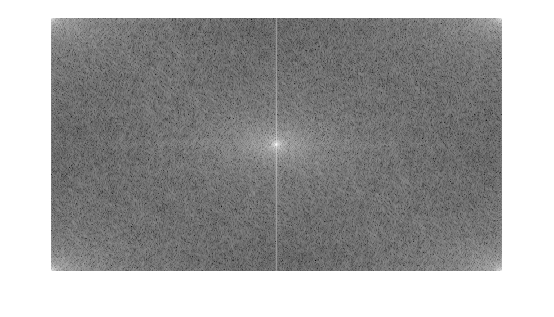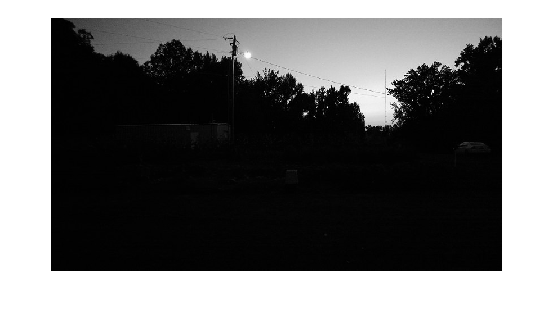## 同态滤波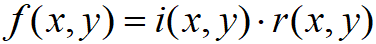?(?,?)在空间上变化缓慢，其频谱集中在低频段，?(?,?)反映图像的细节和边缘，其频谱集中在高频段。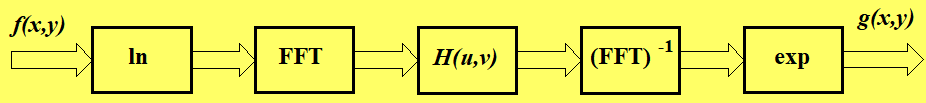?(?,?)?(?,?)=?(?,?)?(?,?)+?(?,?)?(?,?)

?(?,?)=???|?_? (?,?)|=???|?_? (?,?)|∙???|?_? (?,?)|

``````function HF_picture=HF(picture)
picture=double(picture);%对于原图像进行double化，方便后面进行复数运算
LN_picture=log(picture+1);%取对数
LNF_picture=FFT(LN_picture);%进行傅里叶正变换
m=size(picture,1);
n=size(picture,2);
H=zeros(m,n);
D0=10;
rh=1.95;
rl=0.5;
c=1.5;
for i=1:m
for j=1:n
H(i,j)=(rh-rl)*(1-exp(-c*((i-m/2)^2+(j-n/2)^2))/D0^2)+rl;  %同态滤波器的实现
end
end
HFLNF_picture=LNF_picture.*H;  %频域滤波
IFFTHFLNF_picture=IFFT(HFLNF_picture);%傅里叶逆变化
HF_picture=exp(IFFTHFLNF_picture)-1;%在时域取指数，由于最初取对数的时候+1，取指数的时候需要进行减一操作
HF_picture=uint8(HF_picture);%类型转换为八位无符号整数uint8
end
``````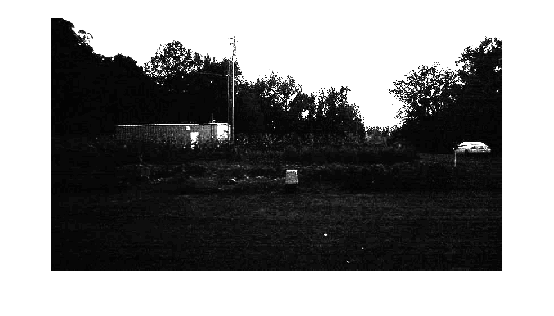``````clc,clear;
picture=rgb2gray(picture);%将图像信息进行灰度化
hist=count_hist(picture);%计算直方图
% stem(H(2,:),H(1,:),'.');%打印直方图
% ylim([0 2*10^4])%限制直方图的Y值的上界限
% imhist(Y)%matlab自带的直方图显示图
[new_picture,new_hist]=hist_equal(picture,hist);
% stem(new_hist(2,:),new_hist(1,:),'.');
% ylim([0 2*10^4])
FFT_picture=FFT(picture);
% imshow(log(abs(FFT_picture)+1),[])
IFFT_picture=IFFT(FFT_picture);

% F1=fft2(picture);
% F1=fftshift(F1);
HF_picture=HF(picture);
imshow(HF_picture)
function hist=count_hist(picture) %计算直方图，输入变量为灰度值，返回矩阵为直方图
Sum=zeros(2,256);%第一行表示的是出现的次数，第二行表示的是0~255供给256个灰度值
for i=1:256
Sum(2,i)=i-1;
end   %第二行的初始化过程
for i=1:size(picture,1)
for j=1:size(picture,2)
Sum(1,picture(i,j)+1)=Sum(1,picture(i,j)+1)+1;%由于灰度图像值是0~255，但索引为1~256，所以索引的时候需要编程X(i，j)+1
end
end  %对第一行进行赋值
hist=Sum;
end

function [new_picture,new_hist]=hist_equal(picture,hist)%直方图均衡化
Y=hist(1,:)/(size(picture,1)*size(picture,2));%将得到的直方图求每个灰度值所对应的概率
for i=2:length(hist(1,:))
Y(i)=Y(i-1)+Y(i);      %这部分是变成概率密度函数，就是进行概率的累加
end
Y=Y*255;%乘以灰度值255
Y=round(Y);%取整
new_picture=ones(size(picture,1),size(picture,2));%建立新的图像
for i=1:size(picture,1)
for j=1:size(picture,2)
new_picture(i,j)=Y(picture(i,j)+1);   %同样，由于灰度图像值是0~255，但索引为1~256，所以索引的时候需要编程picture(i，j)+1
end
end
new_picture=uint8(new_picture);%由于上面的到的类型是double行，imshow的时候需要unit8型的变量
new_hist=count_hist(new_picture);%均衡化后的直方图
end

function FFT_picture=FFT(picture)  %变换并且中心化
G1=zeros(size(picture,1),size(picture,1));   %前变换矩阵
G2=zeros(size(picture,2),size(picture,2));   %后变换矩阵
picture1=double(picture);   %将图像uint8格式转换成double型，便于进行复数相乘
for i=1:size(picture,1)
for j=1:size(picture,2)
picture1(i,j)=picture1(i,j)*exp(complex(0,1)*2*pi*(i+j)/2);%对原图像进行中心化预处理
end
end
for i=1:size(picture,1)   %前变换矩阵的赋值
for j=1:size(picture,1)
G1(i,j)=exp(-complex(0,1)*2*pi*(i-1)*(j-1)/size(picture,1));
end
end
for i=1:size(picture,2)   %后变换矩阵的赋值
for j=1:size(picture,2)
G2(i,j)=exp(-complex(0,1)*pi*2*(i-1)*(j-1)/size(picture,2));
end
end
FFT_picture=G1*picture1*G2;   %二维傅里叶变换并且进行了中心化，返回的是复数型矩阵，要是想进行显示...
%需要在程序中运行   imshow(log(abs(FFT_picture)+1),[])
end

function IFFT_picture=IFFT(f_picture)  %逆变换并且退中心化
G1=zeros(size(f_picture,1),size(f_picture,1));%前变换矩阵
G2=zeros(size(f_picture,2),size(f_picture,2));%后变换矩阵
for i=1:size(f_picture,1)   %前变换矩阵赋初值
for j=1:size(f_picture,1)
G1(i,j)=exp(complex(0,1)*2*pi*(i-1)*(j-1)/size(f_picture,1))/size(f_picture,1);
end
end
for i=1:size(f_picture,2)  %后变换矩阵赋初值
for j=1:size(f_picture,2)
G2(i,j)=exp(complex(0,1)*pi*2*(i-1)*(j-1)/size(f_picture,2))/size(f_picture,2);
end
end
IFFT_picture=G1*f_picture*G2;   %进行逆变换
for i=1:size(f_picture,1)  %退中心化，但是这个时候的到的矩阵还是复数矩阵
for j=1:size(f_picture,2)
IFFT_picture(i,j)=IFFT_picture(i,j)/exp(complex(0,1)*2*pi*(i+j)/2);
end
end
%     IFFT_picture=uint8(IFFT_picture);  %变换成uint8格式，便于之间输出逆变换的图像
end

function HF_picture=HF(picture)
picture=double(picture);%对于原图像进行double化，方便后面进行复数运算
LN_picture=log(picture+1);%取对数
LNF_picture=FFT(LN_picture);%进行傅里叶正变换
m=size(picture,1);
n=size(picture,2);
H=zeros(m,n);
D0=10;
rh=1.95;
rl=0.5;
c=1.5;
for i=1:m
for j=1:n
H(i,j)=(rh-rl)*(1-exp(-c*((i-m/2)^2+(j-n/2)^2))/D0^2)+rl;  %高斯滤波器的实现
end
end
HFLNF_picture=LNF_picture.*H;  %频域滤波
IFFTHFLNF_picture=IFFT(HFLNF_picture);%傅里叶逆变化
HF_picture=exp(IFFTHFLNF_picture)-1;%在时域取指数，由于最初取对数的时候+1，取指数的时候需要进行减一操作
HF_picture=uint8(HF_picture);%类型转换为八位无符号整数uint8
end

``````

2019-07-03 14:00:02 weixin_42902413 阅读数 170
• ###### 携手STM32CubeMX玩转STM32

783 人正在学习 去看看 李凯龙

### 傅里叶变换

傅里叶变换经常被用来分析不同滤波器的频率特性。可以使用2D傅里叶变换（DFT）分析图像的频域特性，实现DFT的一个快速算法被称为快速傅里叶变换（FFT）。

## 1. Numpy中的傅里叶变换

函数np.fft.fft2()可以对信号进行频率转换，输出结果是一个复杂的数组。

• 第一个参数是输入图像，要求灰度图；
• 第二个参数是可选的，决定输出数组的大小；一般输出数组和输入数组一样大，但是如果输出图像比输入图像大，输入图像就需要在进行FFT前补0；如果小，输入图像会被切割。

### 1）将频率为0的部分（直流分量）从图像的左上角移动到图像中心

用np.fft.fftshift()实现，代码如下：

``````import cv2
import numpy as np
from matplotlib import pyplot as plt
f = np.fft.fft2(img)
fshift = np.fft.fftshift(f)
magnitude_spectrum = 20*np.log(np.abs(fshift))
plt.subplot(121), plt.imshow(img, cmap='gray')
plt.title('Input Image'), plt.xticks([]), plt.yticks([])
plt.subplot(122), plt.imshow(magnitude_spectrum, cmap='gray')
plt.title('Magnititude Spectrum'), plt.xticks([]), plt.yticks([])
plt.show()
``````

结果图：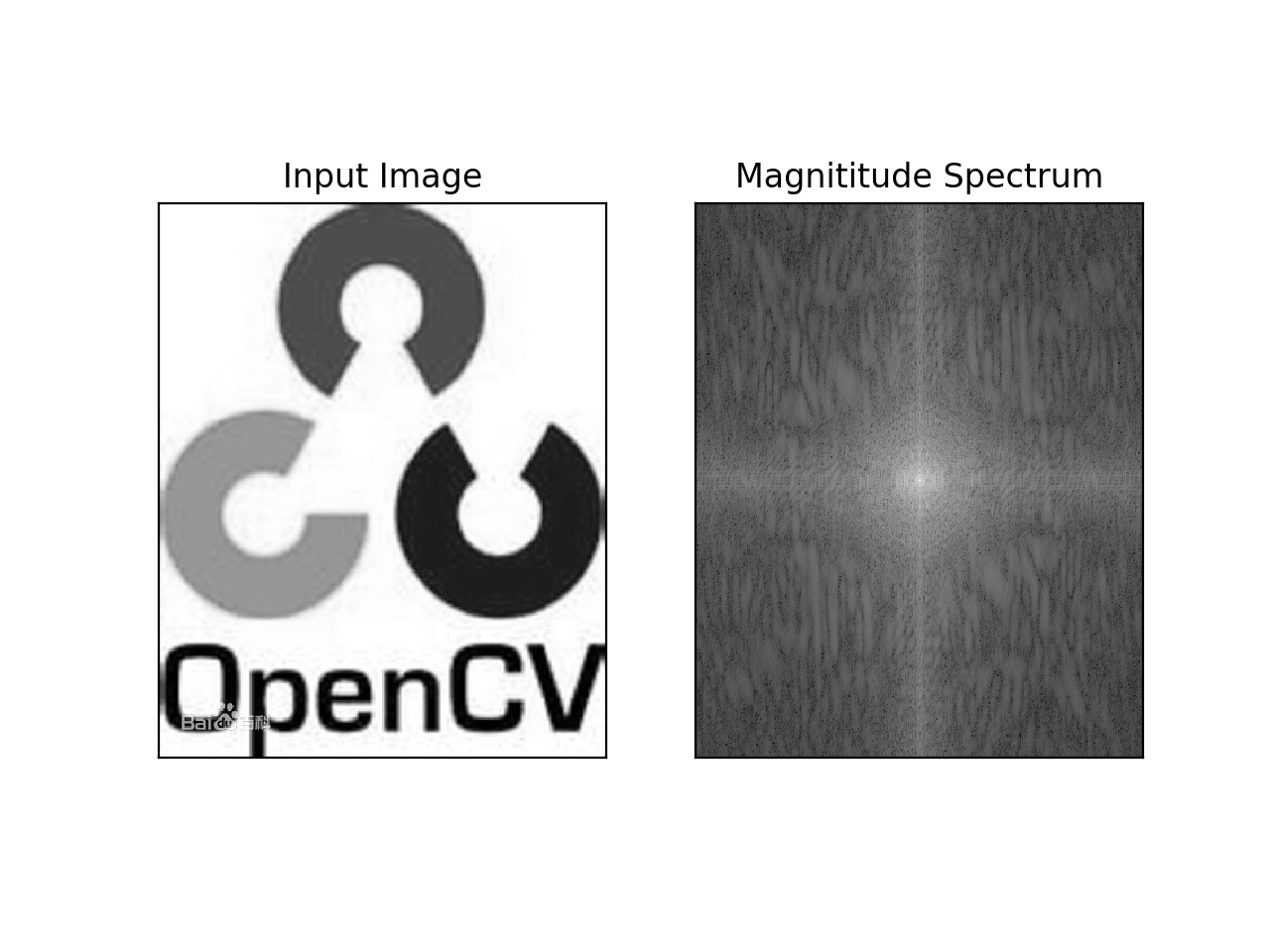### 2）频域变换

使用功能一个60*60的矩形窗口对图像进行掩模操作从而去除低频分量；然后在进行逆平移操作，最后进行FFT逆变换。代码如下：

``````rows, cols = img.shape
crow, ccol = rows//2, cols//2
fshift[crow-30:crow+30, ccol-30:ccol+30] = 0
f_ishift = np.fft.ifftshift(fshift)
img_back = np.fft.ifft2(f_ishift)
#取绝对值
img_back = np.abs(img_back)
plt.subplot(131), plt.imshow(img, cmap='gray')
plt.title('Input Image'), plt.xticks([]), plt.yticks([])
plt.subplot(132), plt.imshow(img_back, cmap='gray')
plt.title('Image after HPF'), plt.xticks([]), plt.yticks([])
plt.subplot(133), plt.imshow(img_back)
plt.title('Result in IET'), plt.xticks([]), plt.yticks([])
plt.show()
``````

结果图：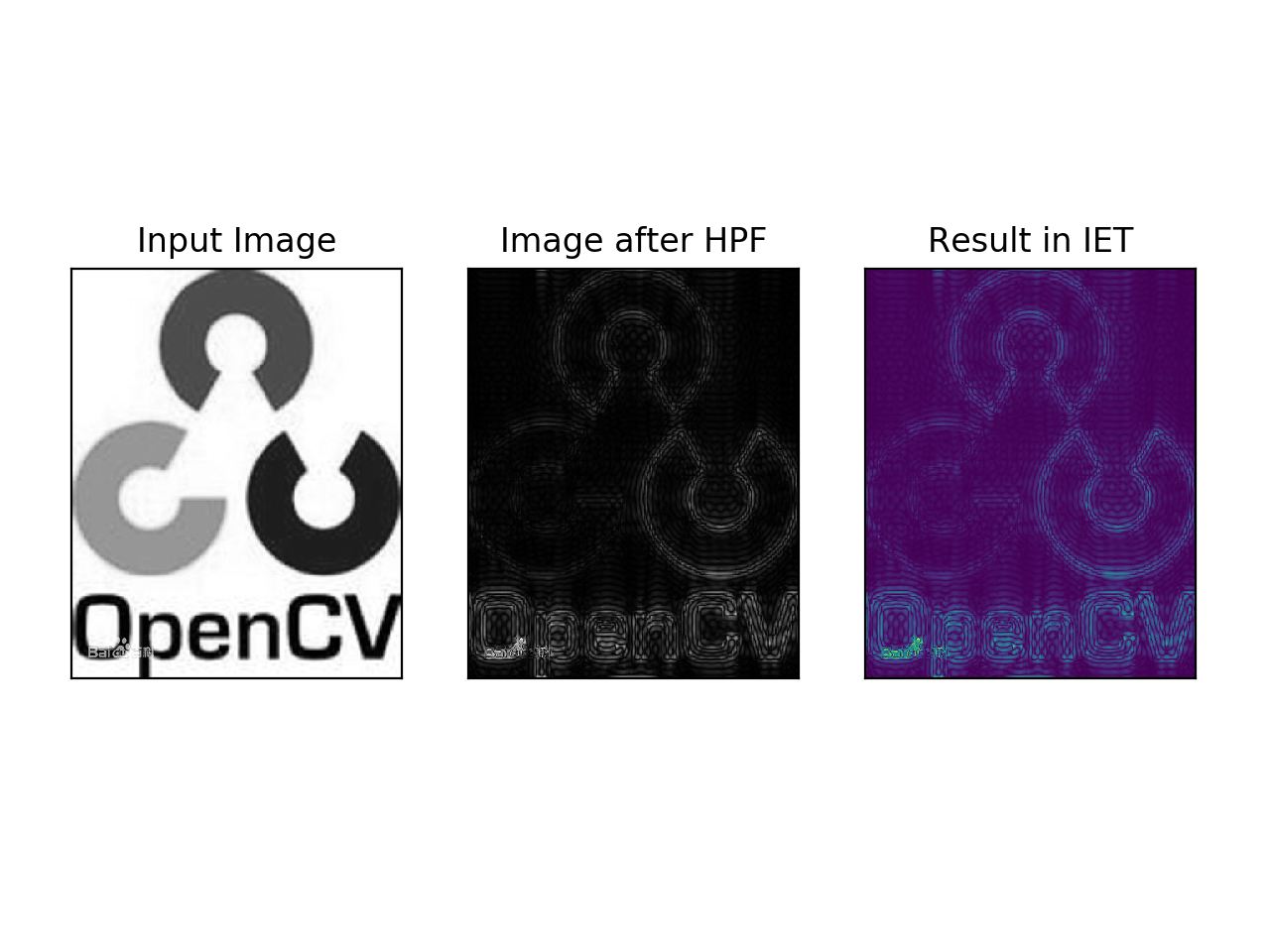从结果图看，高通滤波其实是一种边界检测操作。且由于用矩形窗口滤波出现了振铃效应，所以实际应用中，最好用高斯窗口进行滤波。

## 2. OpenCV中的傅里叶变换

### 1)直流分量平移

``````dft = cv2.dft(np.float32(img), flags=cv2.DFT_COMPLEX_OUTPUT)
dft_shift = np.fft.fftshift(dft)
magnitude_spectrum = 20*np.log(cv2.magnitude(dft_shift[:, :, 0], dft_shift[:, :, 1]))
plt.subplot(121), plt.imshow(img, cmap='gray')
plt.title('Input Image'), plt.xticks([]), plt.yticks([])
plt.subplot(122), plt.imshow(magnitude_spectrum, cmap='gray')
plt.title('magnitude spectrum'), plt.xticks([]), plt.yticks([])
plt.show()
``````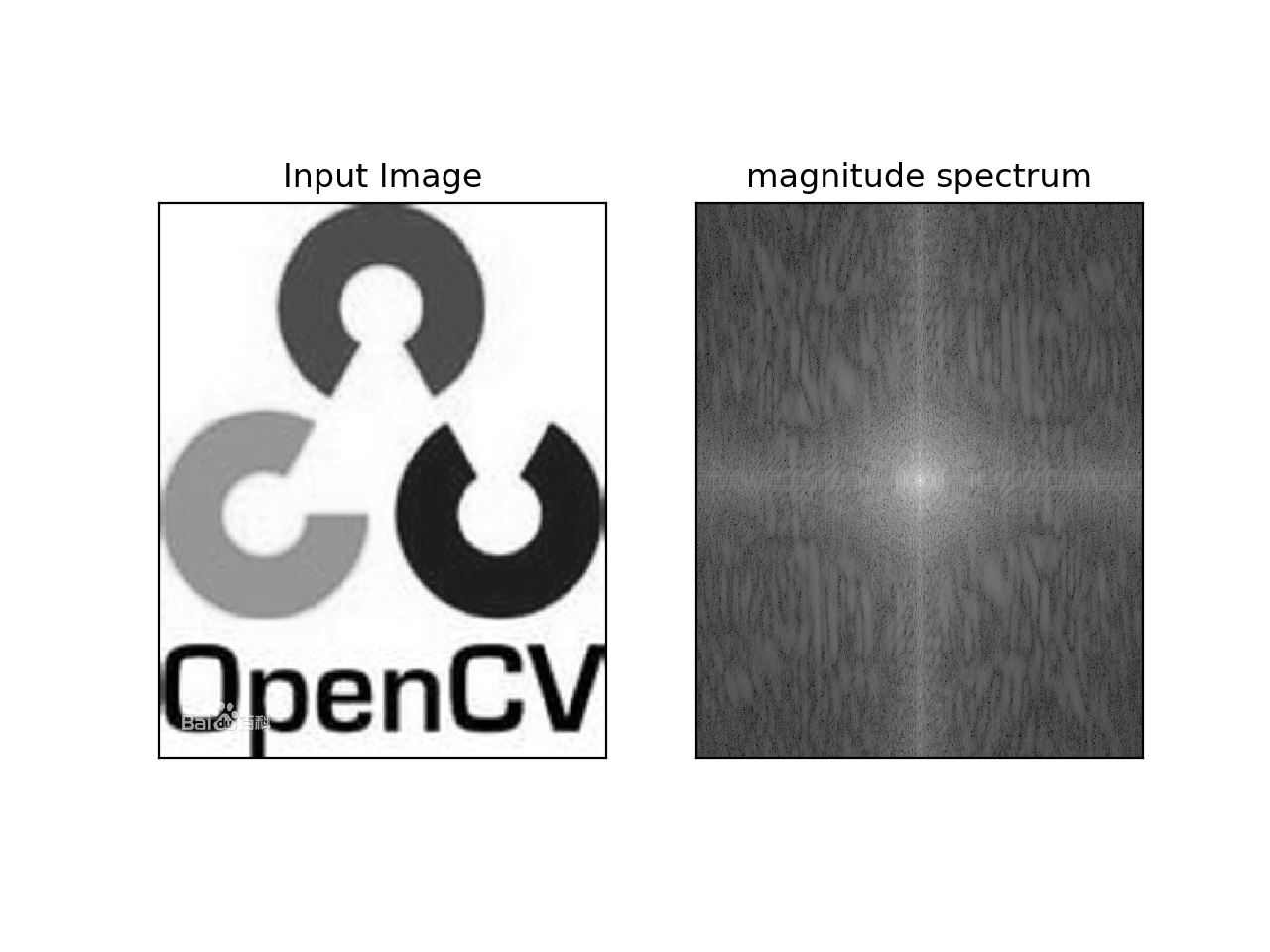### 2）逆DFT

• 先实现HPF（高通滤波）
• 再做LPF（低通滤波）将高频部分去除
``````rows, cols = img.shape
crow, ccol = rows//2, cols//2
#创建掩模，中心部分是1，其余为0
mask = np.zeros((rows, cols, 2), np.uint8)
# 逆DFT
f_ishift = np.fft.ifftshift(fshift)
img_back = cv2.idft(f_ishift)
img_back = cv2.magnitude(img_back[:, :, 0], img_back[:, :, 1])
plt.subplot(121), plt.imshow(img, cmap='gray')
plt.title('Input Image'), plt.xticks([]), plt.yticks([])
plt.subplot(122), plt.imshow(img_back, cmap='gray')
plt.title('img back'), plt.xticks([]), plt.yticks([])
plt.show()
``````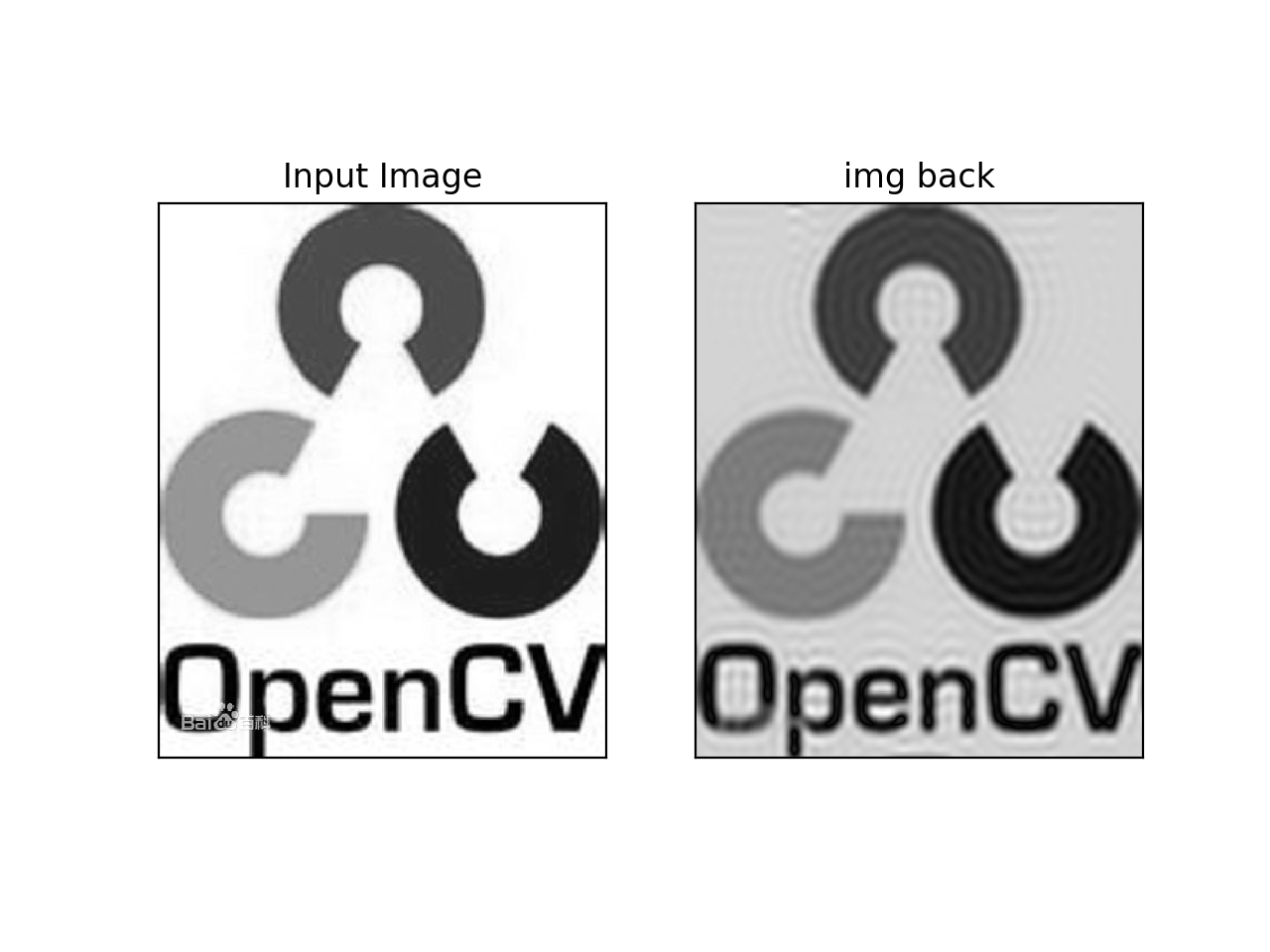cv2.dft() 和cv2.idft()要比Numpy快，但是Numpy更加用户友好。

图像处理中的傅里叶逆变换 相关内容

博文 来自： baidu_41902768

阅读数 513

博文 来自： cyf15238622067

阅读数 15100

阅读数 390

博文 来自： aidem_brown

阅读数 1596

博文 来自： tiemaxiaosu
没有更多推荐了，返回首页